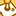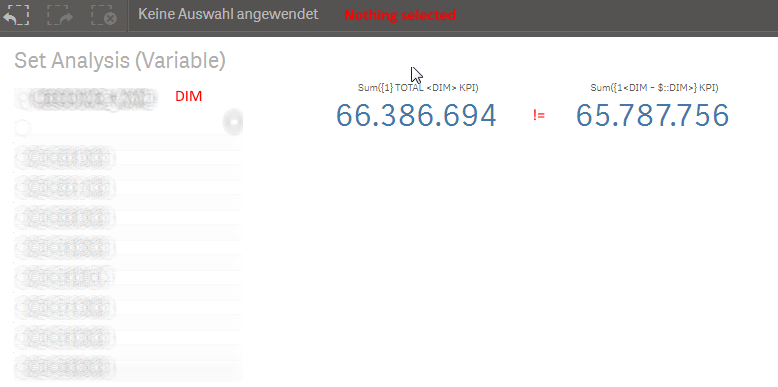# QlikView App Dev

Discussion Board for collaboration related to QlikView App Development.

Announcements
Read about the latest Qlik Community enhancements on the Community News blog!
cancel
Showing results for
Did you mean:Creator

## Set Analysis Sum({1<DIM>}KPI) not equal Sum({\$<DIM=P()>}KPI) if nothing selected

Dear Community,

as i am understanding set analysis correctly

Sum({1<DIM>}KPI) should give the same result (if nothing is selected of DIM) like Sum({\$<DIM=P()>}KPI)

But Sum({\$<DIM=P()>}KPI) shows not the same number, its only nearly (95%) the same as  Sum({1<DIM>}KPI) !

Why is this happening, where is my mistake?

20 RepliesMVP

I am afraid that you are not understanding it correctly.Sum({1<DIM>}KPI) - equivalent to Sum({1} KPI)

Sum({\$<DIM=P()>}KPI) - equivalent to Sum(KPI) unless there is NULL in DIM

Edit:

The NULL consideration is an important case with p() or any explicit mention in the set of the dimension. I.e. here with p() for DIM, only those values would be considered which are not null.MVP

The two expressions are totally different

Sum({1<DIM>}KPI) -> Ignore all selections. You are ignoring selection in DIM twice. This is same as Sum({1} KPI)

Sum({\$<DIM=P()>}KPI) -> Allow all selections. You are allowing for selection in DIM twice. This is same as Sum(KPI)Creator
Author

So here is what i need:

1. Give ALWAYS the total sum of KPI OVER DIM, a selection of any field should not effect the sum

2. Give the sum of KPI OVER DIM, a selection of the DIM field should effect the sumMVP

Is this in a chart? or text box object

1) Sum({1} KPI) or Sum({1} TOTAL <DIM> KPI)

2) Sum({1<DIM = P(DIM)>} KPI) or Sum({1<DIM = \$::DIM>} KPI)Creator
Author

Thanks Sunny for the fast answer, but 2) will not be equal with 1) if DIM is not selected.

We are using a text box object.MVP
 but 2) will not be equal with 1) if DIM is not selected.

Is that a question for me or are you specifying that as a requirement?Creator
Author

We just tested your proposal and its a result of it. Any idea why this is happening?

Tresco mentioned that p() is not considering NULL values, maybe in fact of this?

How can we solve this?MVP

May be like this

2) If(GetSelectedCount(DIM) = 0, Sum({1} KPI), Sum({1<DIM = P(DIM)>} KPI))

or this wasn't working?

Sum({1<DIM = \$::DIM>} KPI)Creator
Author

You are using the same set analysis statements that will not match. The question is not how to show the results, but how to get the first set analysis statement have the same result as the second. While

1. Give ALWAYS the total sum of KPI OVER DIM, a selection of any field should not effect the sum

2. Give the sum of KPI OVER DIM, a selection of the DIM field should effect the sum

we have two textboxes one for 1) and one for 2) and if we clear all selections 1 does not equal 2Community Browser